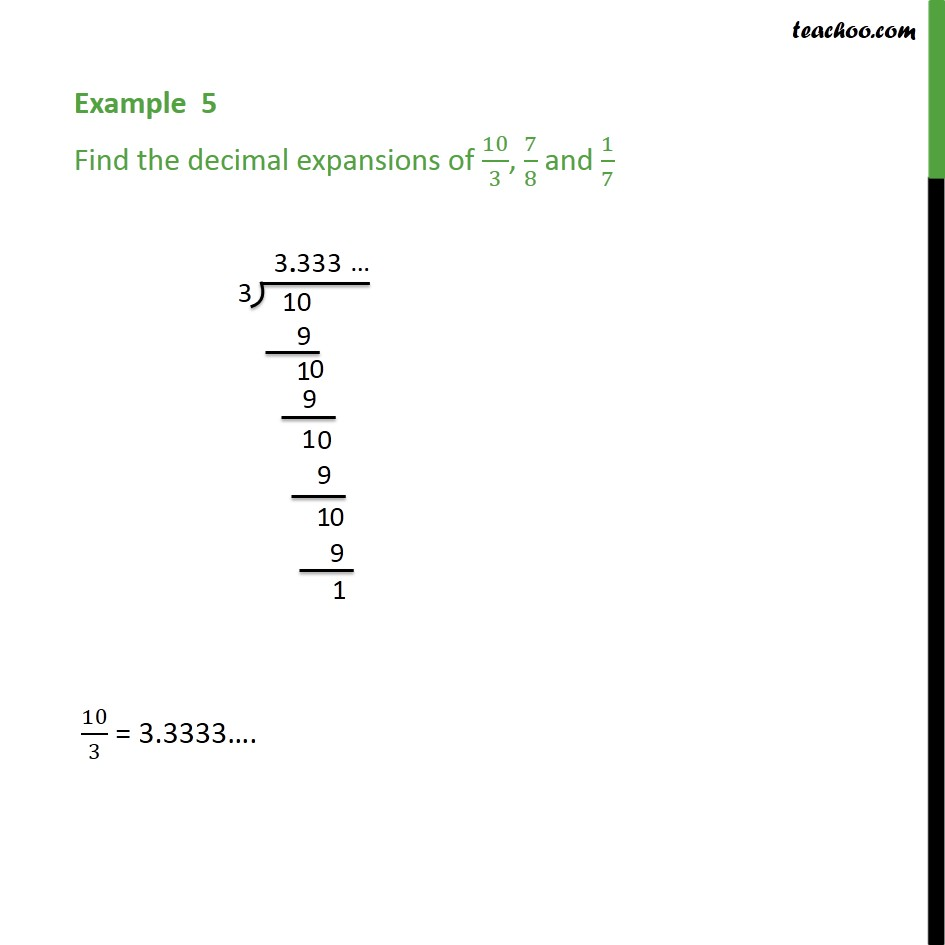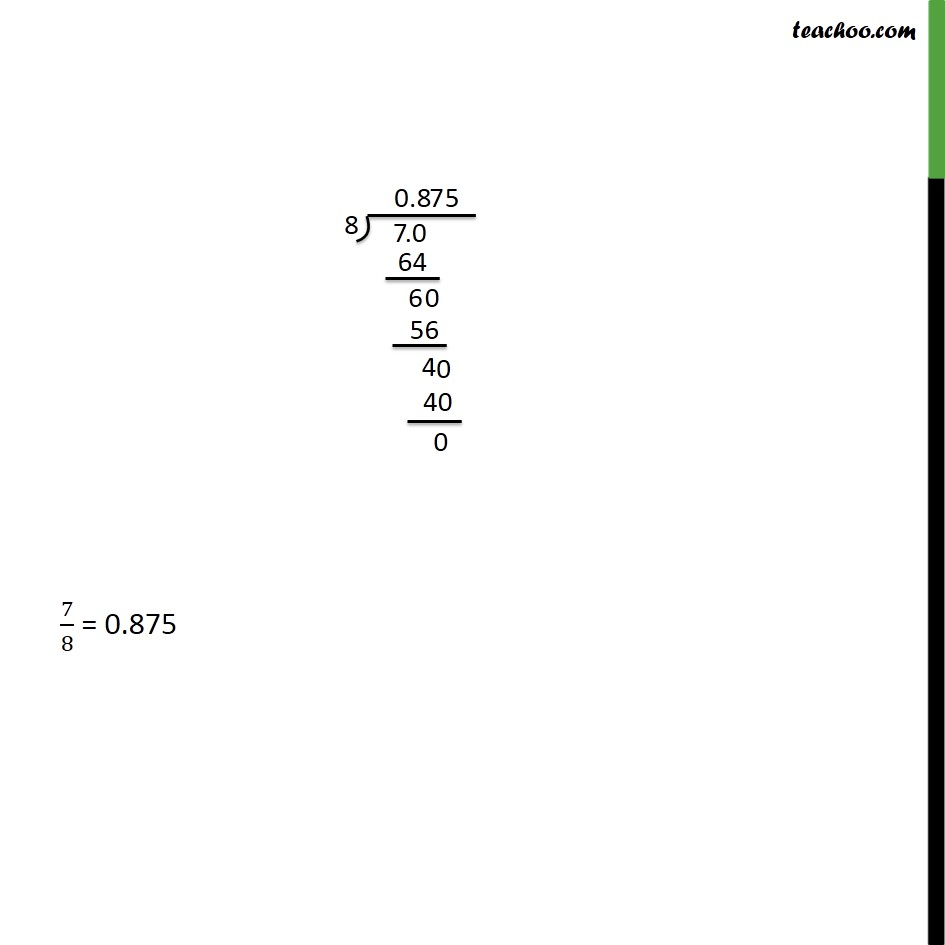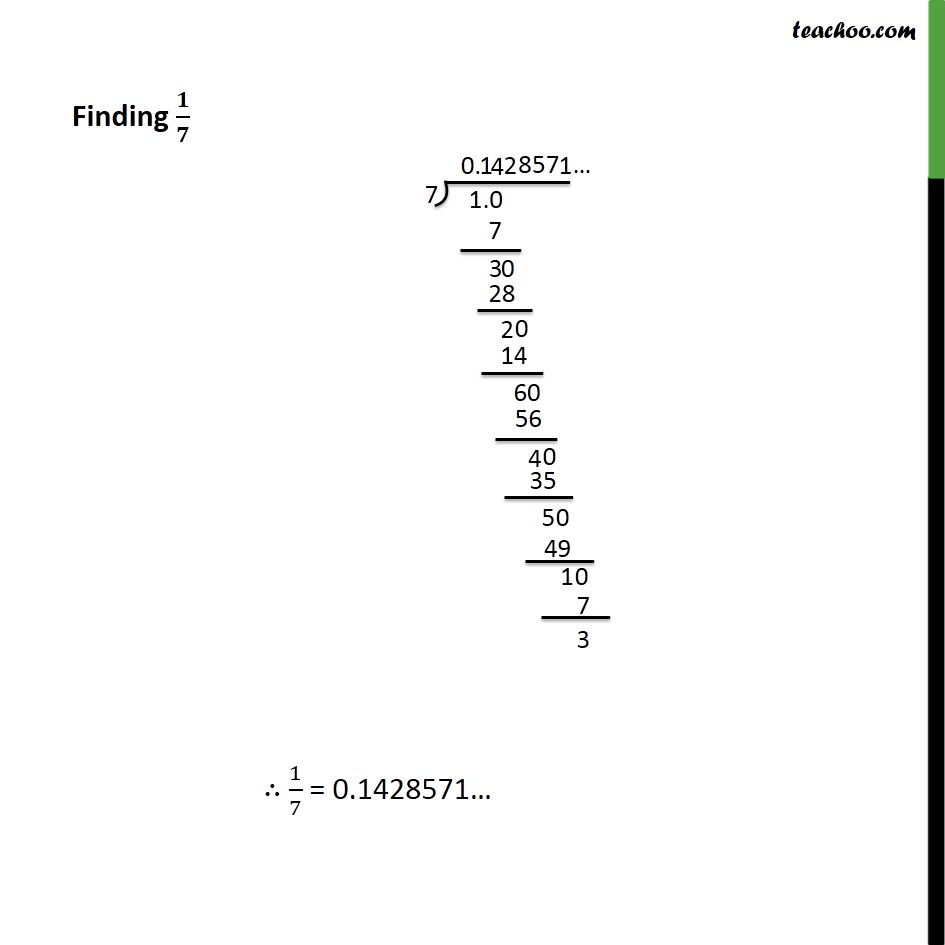1. Chapter 1 Class 9 Number Systems (Term 1)
2. Concept wise
3. Finding decimal expansion

Transcript

Example 5 - Chapter 1 Class 9 Number Systems - NCERT Solution Find the decimal expansions of 10/3, 7/8 and 1/7 To find the Decimal expansions, we divide the numbers Dividing 10 by 3, we get 10/3 = 3.3333 . Dividing 7 by 8 We get 7/8 = 0.875 Dividing 1 by 7 We get 1/7 = 0.1428571... Let's check how we divide. We see that the decimal expansions of the numbers are different